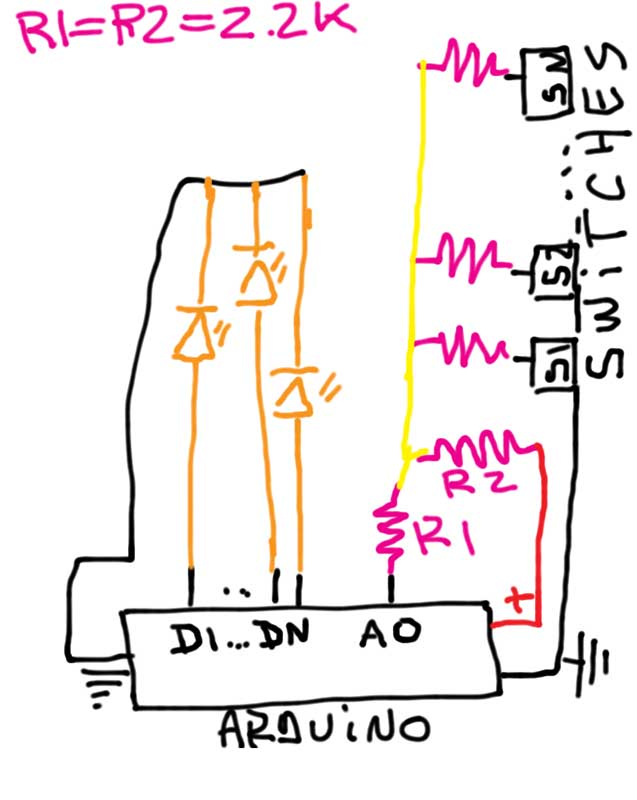# Line 6 pedal board: Programming the Switches and LEDs

After the midi episode, it was time to write the code to get the pedal press signals and control the LEDs for feedback.

The pedal switches use what is called a resistor ladder: each switch is linked to a particular resistor, in series, and in theory, reading the resistor value at the end of the wire lets you guess which pedal was pressed. This particular board is wired in such a way that if you press more than one pedal, only the lowest value registers. This prevents bizarre values from being transmitted to the output wire. But, the engineers at Line 6 decide that ONE particular pair of pedals could be pressed and a distinct resistor value should be passed to the output (pedals associated to switches 6 and 7).Measuring the values was easy with a multimeter. I measured between ground and pin 10 on the 12 pin connector. I wrote the values on the board. Interestingly, pin 9 is also showing the readings, but with 220 Omh added to the readings. Although, sometimes, it’s not 220 Omh but a subtraction! There must be a purpose to this, but it eludes me.

With a bit of trial and error, using a potentiometer to vary the pull up resistor value, I was able to reliably read the values of the resistance associated to each pedal (and the combined press of switches 6 & 7) and write a simple test sketch for the Arduino.

The schematic for the Arduino-Pedal board connection is as follows:The 9 other pins on the connector are linked directly to each of the nine LEDs on the switch board. Each LED has a resistor in series to control current. I was then fairly straightforward to write the code to turn each LED when a particular pedal press is detected.

```/*Line 6 floor board
Connect the resistor ladder from the switches (pedals)
to an analog pin and read the resistor value
Connect the 9 LEDs from the board to separate digital pins

Robert Turenne 2011/04/4

*/
int led1=11;
int led2=12;
int led3=3;
int led4=4;
int led5=5;
int led6=6;
int led7=7;
int led8=8;
int led9=9;
int led10=10;
int swresist=0;
int resistValue;

void setup() {
Serial.begin(9600);
pinMode(led1, OUTPUT);
pinMode(led2, OUTPUT);
pinMode(led3, OUTPUT);
pinMode(led4, OUTPUT);
pinMode(led5, OUTPUT);
pinMode(led6, OUTPUT);
pinMode(led7, OUTPUT);
pinMode(led8, OUTPUT);
pinMode(led9, OUTPUT);
pinMode(led10,OUTPUT);
}

void loop() {

//  Serial.println(resistValue);
if (resistValue < 10)
digitalWrite(led10, HIGH);
else
digitalWrite(led10, LOW);
if (resistValue >= 35 & resistValue < 50)
digitalWrite(led2, HIGH);
else
digitalWrite(led2, LOW);
if (resistValue >= 142 & resistValue < 155)
digitalWrite(led3, HIGH);
else
digitalWrite(led3, LOW);
if (resistValue >= 205 & resistValue < 215)
digitalWrite(led4, HIGH);
else
digitalWrite(led4, LOW);
if (resistValue >= 270 & resistValue < 280)
digitalWrite(led5, HIGH);
else
digitalWrite(led5, LOW);
if (resistValue >= 350 & resistValue < 360)
digitalWrite(led6, HIGH);
else
digitalWrite(led6, LOW);
if (resistValue >= 575 & resistValue < 585)
digitalWrite(led1, HIGH);
else
digitalWrite(led1, LOW);
if (resistValue >= 640 & resistValue < 650)
digitalWrite(led7, HIGH);
else
digitalWrite(led7, LOW);
if (resistValue >= 710 & resistValue < 720)
digitalWrite(led8, HIGH);
else
digitalWrite(led8, LOW);
}```

The code above is for testing purposes, and it worked! Any switch press was decoded and linked to a LED lighting. The values in the “table” were measured using a simple sketch from the standard Arduino environment (Analog Input) and then printed on the terminal window. I chose resistor values, by trial and error, until the readings were spread as much as possible. The values range from 0 to 705, with 966 when no switch is pressed (18.5 K Omh) out of 1024 possible values. I adjusted each “if” statement to allow for a little fluctuation in the reading.  Keeping this interval small gives better timing on switch presses.

The final version of the sketch will use arrays and better logic, with debouncing on the switch presses. This was enough for testing “my” logic.

This entry was posted in Arduino, Pedal board and tagged , . Bookmark the permalink.

### 2 Responses to Line 6 pedal board: Programming the Switches and LEDs

1.Jero Ahumada says:

I’m so happy I found this. I just got a used floorboard for very cheap with the intention of doing exactly this with it.
I was looking at the switches circuit in the pcb and then I found this page and confirmed what I was suspecting, I’ll start doing some tests with your code.
I have one question:
Have you figured out how the expression pedals work? I have no idea, I thought I would find an LED and a photoresistor but instead there are a couple of things that look like a white and yellow LEDs. Do you know how that works? I suspect one emmits and the other receives the light.

•Jero Ahumada says:

Oops, I didn’t see you have other posts with more info. Awesome! Thanks a lot!